# Electric Potential Lesson 39 Electric Potential Consider an

• Slides: 32Electric Potential Lesson 39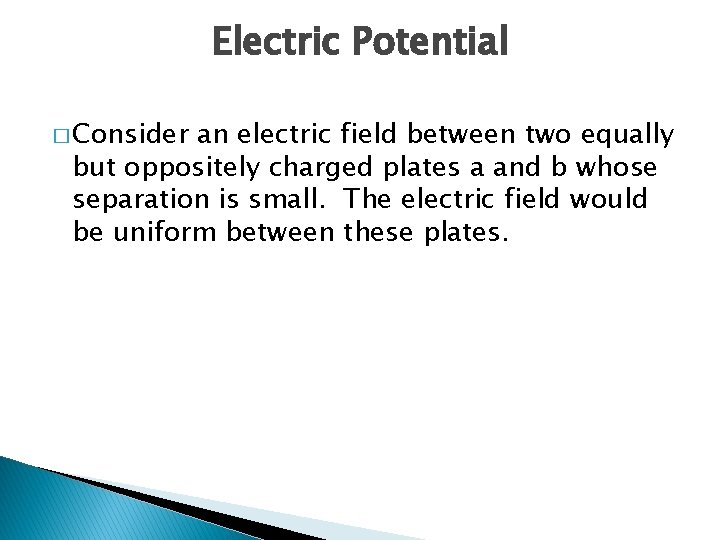Electric Potential � Consider an electric field between two equally but oppositely charged plates a and b whose separation is small. The electric field would be uniform between these plates.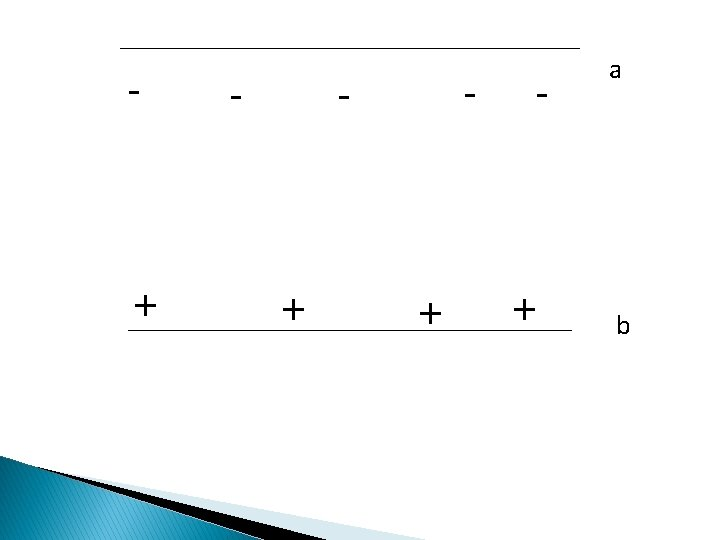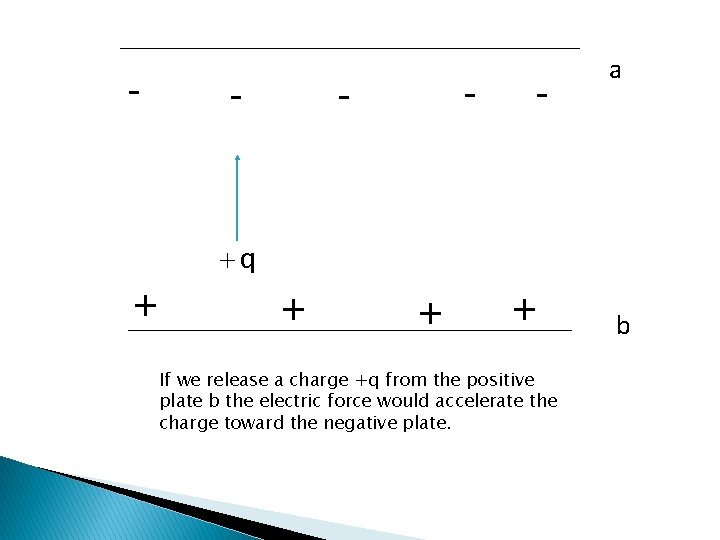- - - a +q + + If we release a charge +q from the positive plate b the electric force would accelerate the charge toward the negative plate. b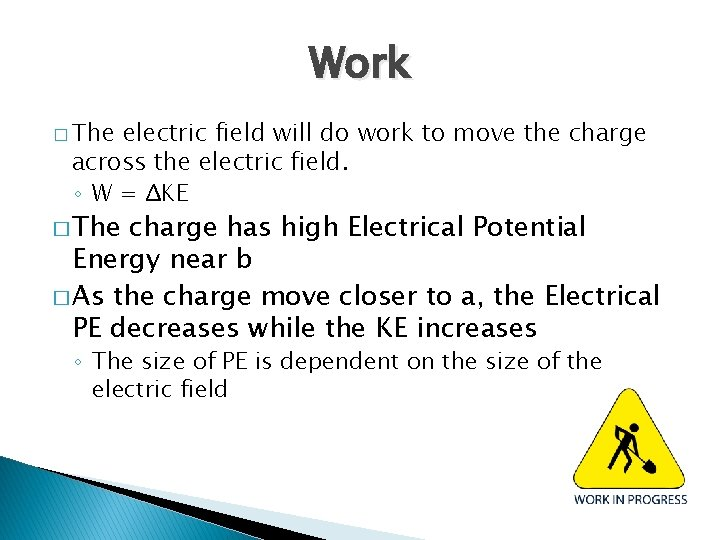Work � The electric field will do work to move the charge across the electric field. ◦ W = ΔKE � The charge has high Electrical Potential Energy near b � As the charge move closer to a, the Electrical PE decreases while the KE increases ◦ The size of PE is dependent on the size of the electric field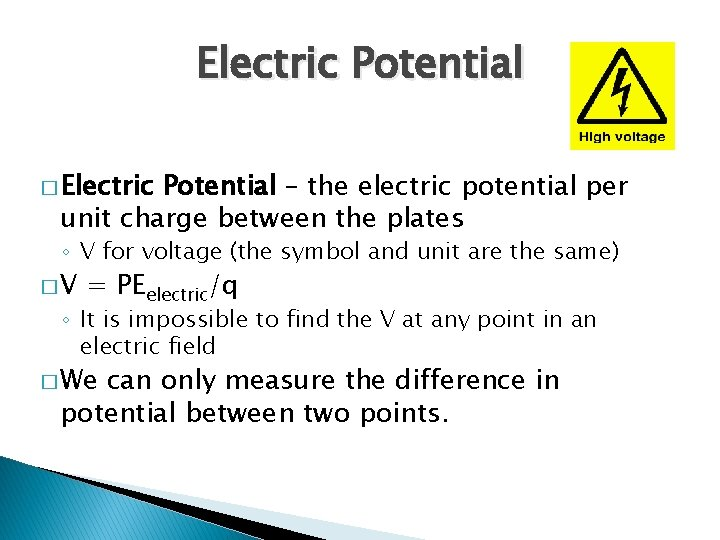Electric Potential � Electric Potential – the electric potential per unit charge between the plates ◦ V for voltage (the symbol and unit are the same) �V = PEelectric/q ◦ It is impossible to find the V at any point in an electric field � We can only measure the difference in potential between two points.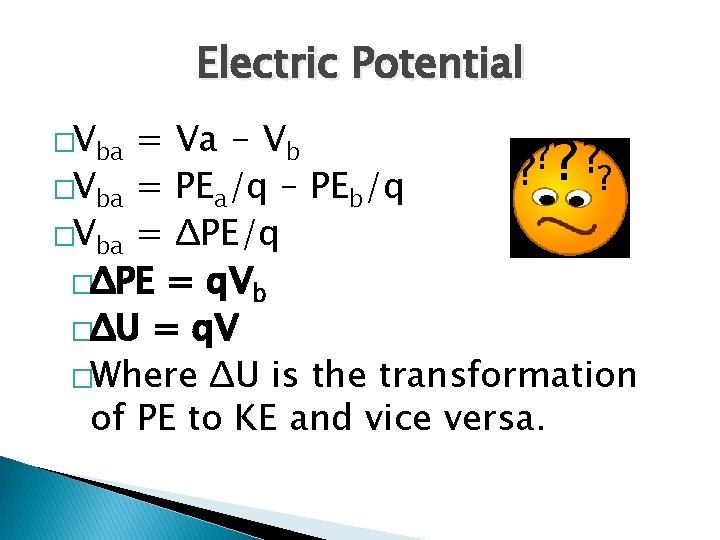Electric Potential �Vba = Va - Vb �Vba = PEa/q – PEb/q �Vba = ΔPE/q �ΔPE = q. Vb �ΔU = q. V �Where ΔU is the transformation of PE to KE and vice versa.Electric Potential and Work � Vba = W/q ◦ where W is the net work to move the charge across the magnetic field. ◦ The unit for voltage is Joules/Coulomb, or simply Volts in honor of Alessandro Volta.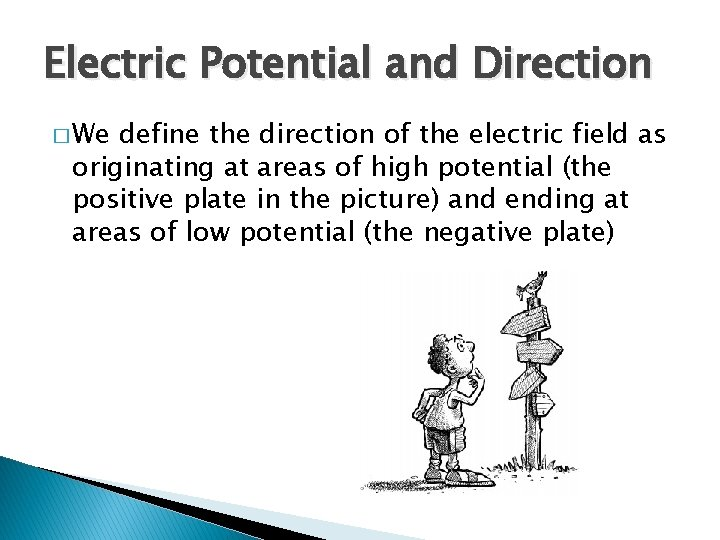Electric Potential and Direction � We define the direction of the electric field as originating at areas of high potential (the positive plate in the picture) and ending at areas of low potential (the negative plate)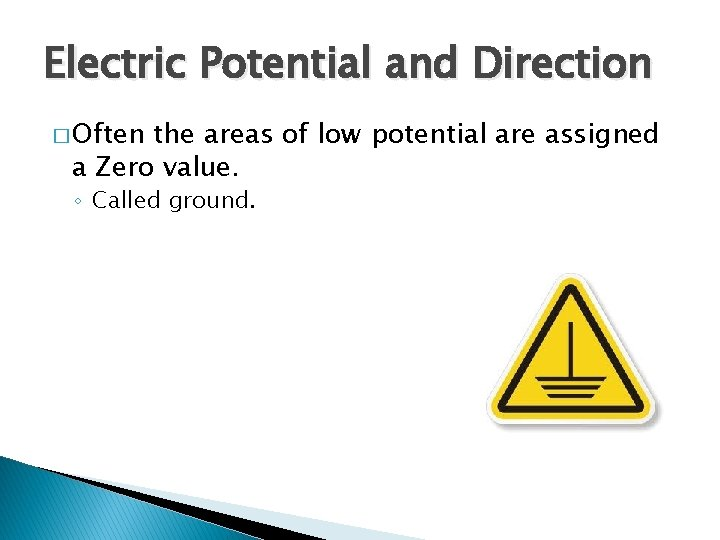Electric Potential and Direction � Often the areas of low potential are assigned a Zero value. ◦ Called ground.Scenario I �A positive charge is moved from the positive plate to the negative plate ◦ Does the charge gain potential (+voltage) or lose it ( -voltage) ◦ Is the change in the PEelectric positive or negative? ◦ Is the work done by the electric field positive or negative?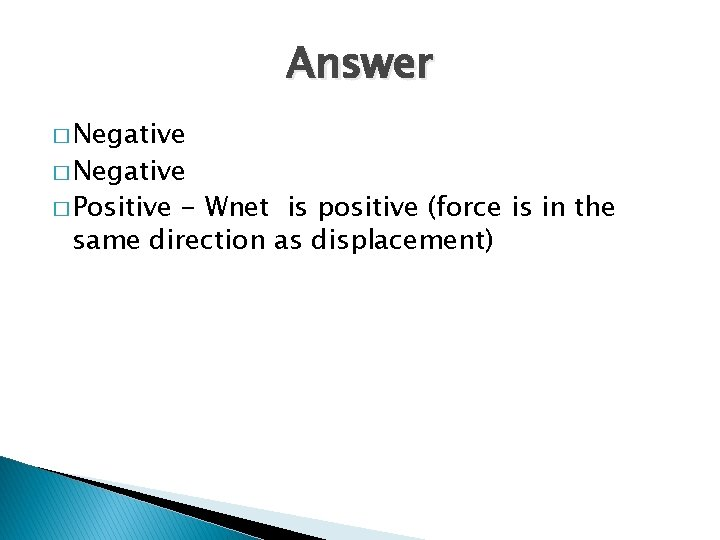Answer � Negative � Positive - Wnet is positive (force is in the same direction as displacement)Scenario II �A positive charge is moved from the negative plate to the positive plate. ◦ Does the charge gain potential (+voltage) or lose it ( -voltage) ◦ Is the change in the PEelectric positive or negative? ◦ Is the work done by the electric field positive or negative?Answer � Positive � Negative(force displacement) is in opposite direction ofScenario III �A negative charge moves from the negative plate to the positive plate. ◦ Does the charge gain potential (+voltage) or lose it ( -voltage) ◦ Is the change in the PEelectric positive or negative? ◦ Is the work done by the electric field positive or negative?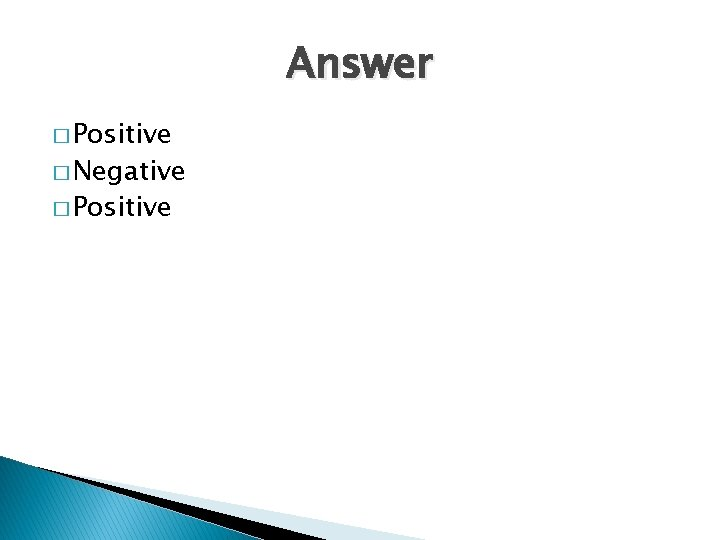Answer � Positive � Negative � PositiveExample I �A charge of – 6. 0μC is moved from ground to a point where the potential is +75 V. a) What is the change in PE? b) Is the work done by the electric field positive or negative? c) How much work is done by the electric field?Answer � -4. 5 x 10 -4 J, Positive, 4. 5 x 10 -4 J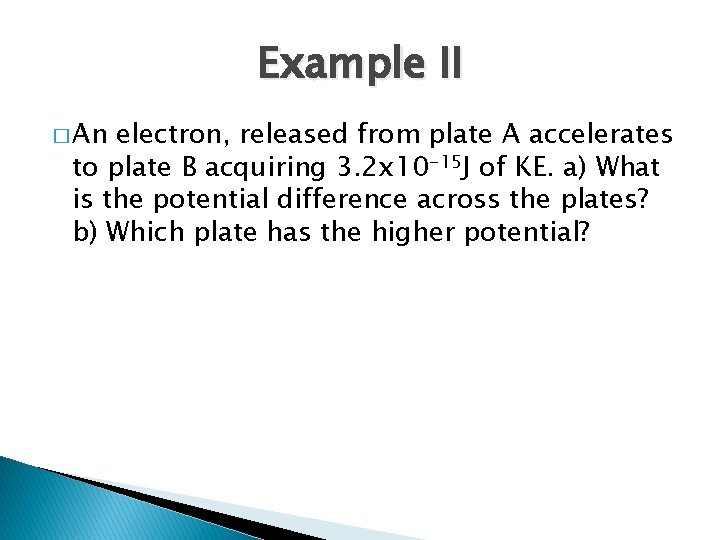Example II � An electron, released from plate A accelerates to plate B acquiring 3. 2 x 10 -15 J of KE. a) What is the potential difference across the plates? b) Which plate has the higher potential?Answer � KE = -PE, 2. 0 x 104 V, B is at a higher potential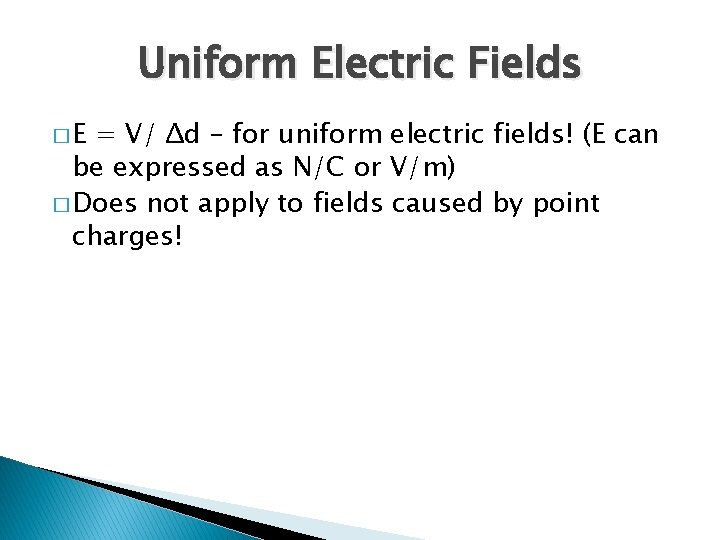Uniform Electric Fields �E = V/ Δd – for uniform electric fields! (E can be expressed as N/C or V/m) � Does not apply to fields caused by point charges!The Electron Volt � The joule is a large quantity of energy when dealing with protons and electrons. The electron volt (e. V) is much smaller. It often provides a more meaningful magnitude for expressing energiesThe e. V � 1 e. V is defined as the amount of energy required to move an electron through a potential difference of 1 Volt ◦ An electron that accelerates through a potential difference of 100 V will lose 100 e. V of electric potential energy and gain 100 e. V of kinetic energy. The e. V is not a proper SI unit so we must convert to Joules when performing calculations involving SI units. ◦ 1. 0 e. V = 1. 6 x 10 -19 JExample III � Two oppositely charged plates A and B are 0. 010 m apart. The E between the plates is 4000 N/C directed from plate B to A. A proton is released from rest at the high potential plate and it accelerates to the low potential plate. a) Determine the voltage difference between the plates. b) Which plate has a higher potential? c) Calculate the kinetic energy, in e. V, of the proton when it reaches the low-potential plate.Answer �V = 40 V, Plate B is high potential, KE = 40 e. VNO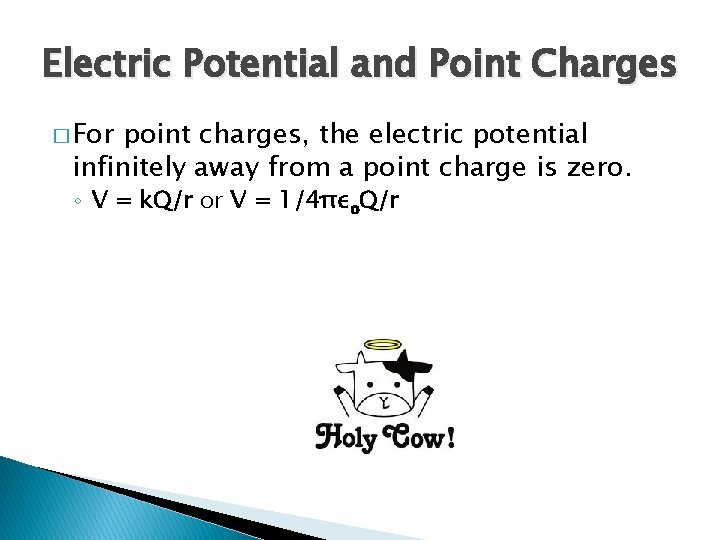Electric Potential and Point Charges � For point charges, the electric potential infinitely away from a point charge is zero. ◦ V = k. Q/r or V = 1/4πεo. Q/r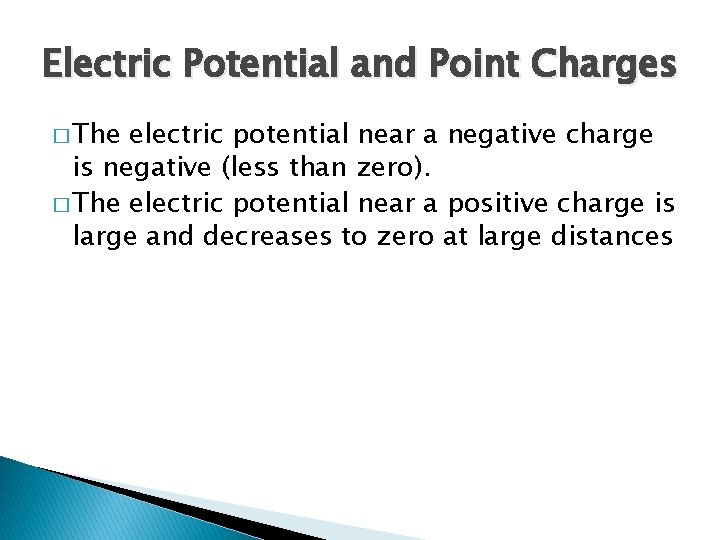Electric Potential and Point Charges � The electric potential near a negative charge is negative (less than zero). � The electric potential near a positive charge is large and decreases to zero at large distances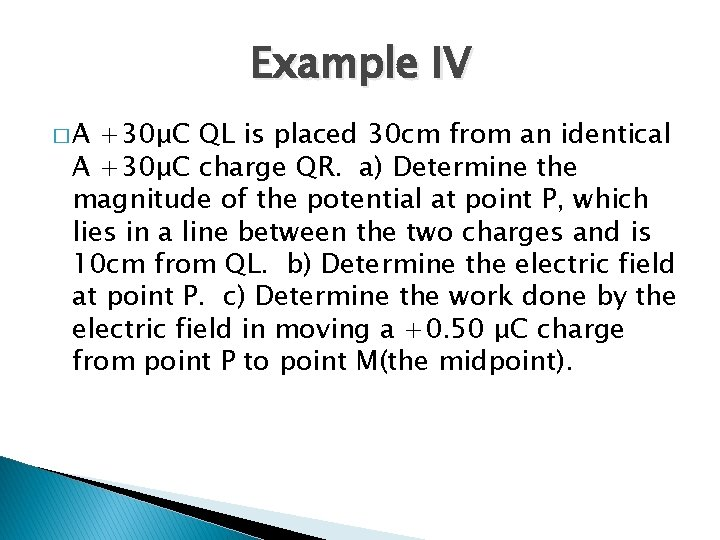Example IV �A +30μC QL is placed 30 cm from an identical A +30μC charge QR. a) Determine the magnitude of the potential at point P, which lies in a line between the two charges and is 10 cm from QL. b) Determine the electric field at point P. c) Determine the work done by the electric field in moving a +0. 50 μC charge from point P to point M(the midpoint).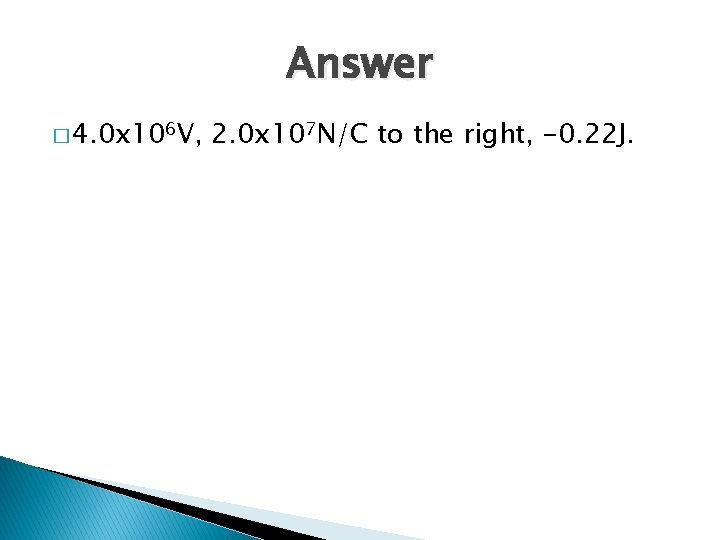Answer � 4. 0 x 106 V, 2. 0 x 107 N/C to the right, -0. 22 J.